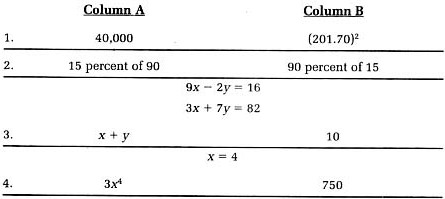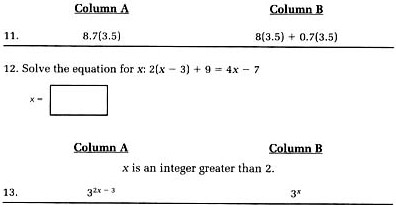# GRE（QUANTITATIVE）模拟试卷14

• 卷面总分：800分
• 试卷类型：历年真题
• 测试费用：5.00 学币
• 答案解析：有
• 练习次数：3次
• 作答时间：30分钟
• 关注人数：849

### 试卷简介

#### 题型：

• 1.
• 2.
• 3.1

Directions for Quantitative Comparison Questions: Some of the following questions give you two quantities, one in Column A and one in Column B, Compare the two quantities and choose one of the following answer choices:

A. if the quantity in Column A is greater;

B. if the quantity in column B is greater;

C. if the two quantities are equal;

D. if you cannot determine the relationship based on the given information(1)

A．

B．

C．

D．

2

(2)

A．

B．

C．

D．

3

(3)

A．

B．

C．

D．

4

(4)

A．

B．

C．

D．

5

Directions for Quantitative Comparison Questions: Some of the following questions give you two quantities, one in Column A and one in Column B, Compare the two quantities and choose one of the following answer choices:  A. if the quantity in Column A is greater;

B. if the quantity in column B is greater;

C. if the two quantities are equal;

D. if you cannot determine the relationship based on the given information(5)

A．

B．

C．

D．

6

(6)

A．

B．

C．

D．

7

(7)

A．

B．

C．

D．

8

Directions for Quantitative Comparison Questions: Some of the following questions give you two quantities, one in Column A and one in Column B, Compare the two quantities and choose one of the following answer choices:  A．if the quantity in Column A is greater;

B．if the quantity in column B is greater;

C．if the two quantities are equal;

D．if you cannot determine the relationship based on the given information(8)

A．

B．

C．

D．

9

(9)

A．

B．

C．

D．

10

(10)

A．

B．

C．

D．00****08   2013-11-20 03:24:3800****41   2013-11-12 17:01:1500****50   2013-06-27 17:58:13

### 其他考生还关注了这些

• #### GRE（QUANTITATIVE）练习试卷16

##### 5 人做过2155人关注### 下载试卷